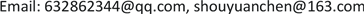1北京师范大学天文系，北京

2山东师范大学，信息科学与工程学院，山东 济南1. 天文信号处理模式发展过程

V f = H C × D (1)

2. 信道天文学：天文信号的信道模式处理

Y ( x , t ) = H ( x , t ) * s ( t ) + n ( t ) (2)

s ( t ) = y ( x , t ) − n ( t ) H ( x , t ) (3)

3. 宇宙红移的信道模式处理

s ( t ) = A sin ϖ t (4)

I ( x ) = I ( x 0 ) e − 2 α x (5)

A ( x ) = A ( x 0 ) e − α x (6)

f o = f s e − α f x ； λ o = λ s e − α f x (7)

Z = λ o − λ s λ s (8)

Z = λ o − λ s λ s = e α f x − 1 (9)

Z = e α f x − 1 ≈ α f x (10)

1 Mpc = 106 pc = 3.2616 * 106 l.y. = 3.2616 * 106 * 0.94607 * 1016 m = 3.08568 * 1022 m；

H1 m = 0.8 * 10−26/m (11)

Z = λ o − λ i λ i ≈ f i − f o f i (12)

∇ f = f i − f o ≈ f i * Z = Z * D * f i (13)

Δ θ = 2 π * Δ t * Δ f = 2 π * Δ t * Z * f i = 2 π * Δ t * H D * f i (14)

Δ θ = 360˚ * 86400 s * 0.8 * 10−26/m * 2 * 104 m * 1015 Hz/s = 5˚(15)

4. 超新星信号的信道模式处理

a n d n y ( t ) d t n + ⋯ + a 0 y = b m d m x d t m + ⋯ + b 0 x (16)

δ ( t ) = [ ∞ , t = 0 0 , t ≠ 0   与   ∫ − ∞ + ∞ δ ( t ) d t = A (17)

a 2 d S o 2 d t 2 + a 1 d S o ( t ) d t + a 0 S o ( t ) = b 0 S i ( t ) (18)

H ( S ) = b 0 a 2 s 2 + a 1 s + a 0 (19)

1) 临界阻尼状态响应：

S o ( t ) = b 0 a 2 * t * e − a 0 a 2 * t (20)

2) 过阻尼状态

S o ( t ) = ϖ 0 k 2 ξ 2 − 1 [ e − ( ξ + ξ 2 − 1 ) ϖ 0 t − e − ( ξ − ξ 2 − 1 ) ϖ 0 t ] (21)

3) 欠阻尼状态

S o ( t ) = ϖ 0 k 2 1 − ξ 2 [ e − ξ ϖ 0 t sin ( 1 − ξ 2 ϖ 0 t ) ] (22)

S o ( t ) = 0.41 t * e − 0.05977 t (23)

2011年诺贝尔获奖者成果：发现超新星亮度比预期的低、红移比预期的大

4.1. 亮度比预期低的信道解释

M − m = − 2.5 log ( F 10 F D ) = 5 − 5 log d ( p c ) (24)

S o ( t ) = b 0 a 2 * t * e − a 0 a 2 * t

4.2. 红移比预期的大

λ o = λ s e α f d

Z = λ o − λ s λ s = e α f d − 1 ≈ α f d

S o ( t ) b 0 a 2 * t * e − a 0 a 2 * t

5. 信道对波的弥散作用

6. 结论